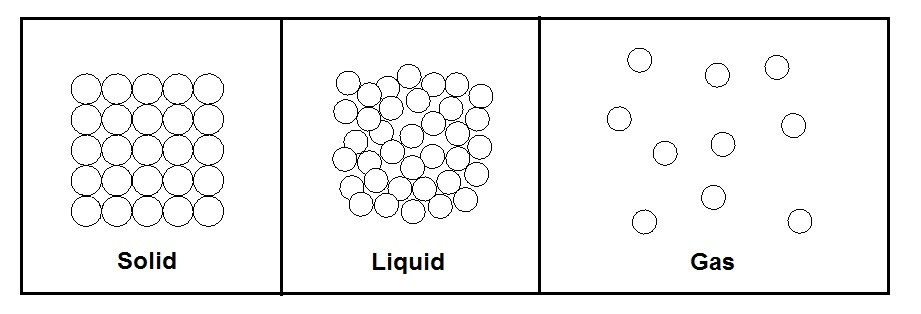# Temperature

When talking about temperature, most people refer to it as a measurement of hotness or coldness.  However, in reality it is not easy to give an exact definition to it.  The reason why is because what truly is hot and cold.  One person might feel cold while another is thinks it just right.

Instead of referring to temperature as hot and cold you need to think about what is actually being measured.  In reality when you are measuring temperature you are actually measuring the kinetic energy with the molecular bonds between atoms. As a result, the “colder” a molecule is the lower the kinetic energy.  On the other hand, the “hotter” a molecule is the higher the kinetic energy.

### Temperature and Phase

The three main phases of matter are solid , liquid, and gas.  Temperature as well as pressure has a direct effect on the phase the matter is in.  However, let’s take a look at the phases of matter at a molecular level.

The phase that matter is in is directly related to bond strength vs the kinetic energy within the molecule.  First let’s take a look at a solid.  When matter is in a solid state the bond strength is significantly strong enough to overcome the kinetic energy within the matter.  As a result, the atoms are held tightly together.  There is however still molecular vibration between the bonds due to the kinetic energy.

Next, let’s take a look at the liquid form of matter.  During the phase there is enough kinetic energy within the molecule that the molecules will start to flow over each other.  There is, however, still enough bond strength to loosely keep the molecules together.  As a result, a fluid will take the shape of its container but will keep its volume.  A liquid is not compressible.

Finally, the last state of matter to consider is gas.  In this state the kinetic energy of the molecules has completely overcome the bond strength of the molecules.  As a result, the molecules will bounce around randomly within its container.  In addition, they will also expand or contract to take the shape of the container they are in.   Unlike a liquid a gas it compressible.

The figure below shows the three phases of matter at the molecular level.### Temperature Scales

The two temperature scales that are most commonly used are the Celsius scale and the Fahrenheit scale.  The Celsius scale is the standard international system of measurement (SI System) for temperature. At 0 degrees C water will start to freeze, and at 100 degrees C water will boil.  Fahrenheit on the other hand is used to measure temperature in the English system of measurement. For Fahrenheit water will freeze and 32 degrees and will boil at 212 degrees. To relate the two scales to each other the following equation would be used.

(Eq 1)  $T(^oF)=1.8T(^oC)+32$

However, in thermodynamics the thermodynamics scales are used. The thermodynamic scales do not have negative number values, but instead define an absolute zero. At absolute zero there will be no kinetic energy present between molecular bonds. This value has never been reached in real life, but scientists have come close. The thermodynamics scales are Kelvin and Rankine. The Kelvin scale is SI thermodynamic scale and can be related to the Celsius scale using the following equation.

(Eq 2)  $T(K)=T(^oC)+273.15$

The Rankine scale, on the other hand, is the English thermodynamic scale. To relate the Fahrenheit scale to the Rankine scale the following equation would be used.

(Eq 3)  $T(R)=T(^oF)+459.67$

When solving thermodynamic problems the thermodynamic scales should be used.

### The Zeroth Law of Thermodynamics

The Zeroth Law of Thermodynamics is one of the fundamental laws of thermodynamics. It is called the zeroth law because it came after the 1st and 2nd law of thermodynamics when in fact it should have come before. What the Zeroth law says is “If two bodies are in thermal equilibrium with a third body then they are in equilibrium with each other.” In other words all bodies within the system must have the same temperature otherwise there will be have to be some sort of energy transfer between the objects until they reach an equilibrium state.